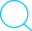# 汉明码解析

## 计算机组成原理

Posted by Kingtous on November 30, 2019

#### 举例：

• 求汉明码

0101按偶配置的汉明码

• 根据公式1得：k=3

• 1 2 3 4 5 6 7
C1 C2 0 C3 1 0 1

要保证偶配置，则需要让相应位的1的数量为偶数

原则:

• C1: 包含1,3,5,7,9,11…
• C2: 包含…(怎么求呢？向下看↓)
1. 每个小组$g_i$有一位且只有一位独占，那位为第$2^{i-1}$位
2. 每两个(注意是每两个，不只是相邻的)$g_i$和$g_j$共享独占的一位，其他的组都没有，为第$2^{i-1}+2^{j-1}$位
3. 每三个…
4. 每四个…
• 纠错（只能纠正一位）

背景：按偶配置

• 每一组的异或值应该为0，否则存在位数错误

假设收到偶配置的0100111

$P_0 = 0(d_1) \oplus 0(d_3) \oplus 1(d_5) \oplus 1(d_7) =0$

$P_1 = 1(d_2) \oplus 0(d_3) \oplus 1(d_6) \oplus 1(d_7) = 1$ 此处有问题

$P_2 = 0(d_4) \oplus 1(d_5) \oplus 1(d_6) \oplus 1(d_7) = 1$此处有问题

$P_0$为0，说明第1，3，5，7位没问题，$P_1$和$P_2$有问题，而纠错默认只有1位错误，所以$d_6$出现问题，把$d_6$纠正为0，纠错完成